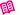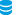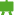Skrýt# Frequency

In corpus linguistics, frequency is the number of times a given form or phenomenon occurs in the corpus. It is either given as an absolute value, e.g. the lemma pes occurs in the 100 million word corpus SYN2010 17 701 times, or as a relative value, e.g. the lemma pes occurs in SYN2010 (after taking into account the varying number of words and positions in the corpus) 145 times per million words (the abbreviations used are ipm = instances per million or ppm = parts per million).

While the absolute frequency (i.e. the number of a word's occurrences in the corpus) requires further specification (the total size of the corpus or the frequency of another phenomenon for comparison), relative frequency (i.e. absolute frequency in proportion to the total size of the corpus) in and of itself serves to show the frequency of the phenomenon and makes it possible to compare corpora or texts of varying sizes.

The relative frequency (REL), based on the total size of the corpus (N), is calculated using the absolute frequency (ABS) with the formula:The relative frequency in such cases is at the same time an estimate of the probability of the given phenomenon in the language (times 1 million).

Because frequency is intuitively and introspectively inaccessible, corpora are the main source of information about it. Simultaneously, in corpus linguistics frequency is considered to be a basic indicator which has a crucial influence on the description of language and the evaluation of the nature (and importance) of a given form or phenomenon.

## Rank

Rank is another way of relativizing frequency. In a list of phenomena sorted by frequency, we assign rank 1 to the phenomenon with the highest frequency, rank 2 to the phenomenon with the second highest frequency, etc. Rank n, where n is the total number of items on the list, is assigned to the phenomenon with the lowest frequency. Just like frequency, rank can also be relative (sometimes labelled rr), and it is calculated according to the formula:,

where n is the number of types in the corpus.

## Measured and expected frequency

Aside from the values returned for the individual phenomena in the corpus, we also work with values which we can expect in the corpus based on external information (e.g. previous research conducted on different data). It is the difference between the values O (observed) and E (expected). By examining these indicators we can discover whether or not the given phenomenon is noticeably or unusually frequent in the corpus, which can lead to the identification of some specific phenomena (e.g. collocations, keywords etc.).

If we know the probability of a word's occurrence, we can use a simple formula to find the expected frequency of the given word in a corpus of a specified size.where:

• p(A) is the probability of word A
• N is the size of the corpus in numbers of tokens

We will never know the exact probability of the phenomenon in a population of all manifestations, but it can be approximated by the relative frequency discovered in previous comparisons using different data (other corpora). In the SYN2005 corpus we can therefore determine the probability of the occurrence of the lemma škola ('school') from its frequency (f = 47872) and from the total size of the corpus (N = 122419382):Based on this probability we can calculate the expected frequency of the lemma škola in the corpus SYN2010 (N = 121667413).By searching the SYN2010 corpus we can easily find the actual frequency of this lemma:The measured and expected values can then be compared, e.g. with the aid of the chi2 test.

## The use and significance of frequency

Frequency as a fundamental value of an arbitrary unit (type) and langue (system) characteristic is used not only for determining the relations between alternating phenomena (e.g. the frequency of morphological variants bychom and bysme, as in SyD), but it also serves the compilation of dictionaries (defining the most frequent words as core vocabulary), the extraction of collocations, the evaluation of grammatical categories, the identification of keywords in texts etc.

In order to interpret frequency correctly it is necessary to realize that it is a point estimate of the frequency of phenomena in the entire language. Every corpus is more or less a precise approximation of the population in question (=texts of a certain nature), and therefore in different corpora created using the same methodology (even if it were possible to guarantee their full comparability) the frequencies of the desired phenomenon will differ slightly. This variability can be captured using the confidence interval which gives the span containing (with a certain probability) the frequency of a given phenomenon. For finding out the confidence interval we use a binomial distribution, the input values being the frequency of the phenomenon, the size of the corpus and the significance level (expressing a tolerable error rate).

The confidence interval around the measured frequency on the significance level of 0.95 says that in an experiment which would encompass an infinite number of comparable corpora of the same size, the frequency of the given phenomenon would be within this interval in 95% of measurements. When conducting our analysis we should always be aware that the actual frequency of a phenomenon can acquire any value from the confidence interval.

#### Examples

If we measure in a corpus of 100 mil. words (e.g. SYN2015) 50 occurrences for a given phenomenon, the results must be interpreted that in a population of texts which the corpus strives to represent, this phenomenon appears in the range from 37 to 66 occurrences per 100 mil. words (with a 5% error rate, i.e. with the risk that the actual result will be found outside the given interval).

If we discover that the given phenomenon occurs in a corpus (e.g. in ORAL2008) exactly three times, it means that in another fully comparable corpus the same could have an occurrence rate of up to 9 hits, or it could be absent completely (again with a 5% error rate).1)

## Dispersion of phenomena

In some cases it is necessary to supplement absolute or relative frequency with information about the dispersion of the given phenomenon throughout the text/corpus. Even phenomena which are relatively very frequent can appear only in a limited circle of texts or in certain parts of the document. In such cases, the frequency itself can be an unreliable indicator of conventionality. In order to quantify the uneven distribution of words in corpora, various measures of dispersion are used, the most simple of which are based on counting the number of documents in which the unit appears, or authors who used it. More sophisticated ways of obtaining information about dispersion include using average partial frequencies within individual sections of the text/corpus, or calculating the variation coefficient i.e. the ratio of the standard deviation of frequencies in the individual sections to the average of these partial frequencies (e.g. Juilland's D coefficient, see also ARF).

1)
Such low values also depend on the selected rounding up strategy.
•KonText manual
•CNC corpora
•Basic course